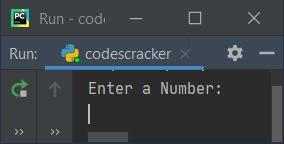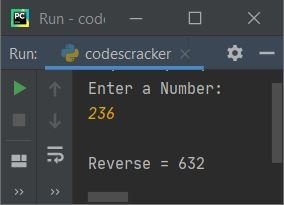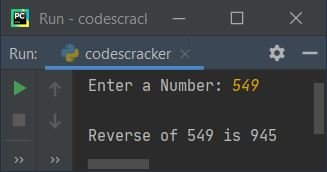# Python Program to Reverse a Number

This article is created to cover some programs in Python, that find and prints reverse of a number entered by user. Here are the list of approaches used:

• Find reverse of a number using while loop
• Using for loop
• Using list
• Using function
• Using recursion

## Reverse a Number using while Loop

To find reverse of a given number in Python, you have to ask from user to enter a number, then reverse and print like shown in the program given below. The question is, write a Python program to reverse a number. Here is its answer:

```print("Enter a Number: ")
num = int(input())

rev = 0
while num!=0:
rem = num%10
rev = rem + (rev*10)
num = int(num/10)

print("\nReverse =", rev)```

Here is its sample run:Now supply the input say 236 as number, press `ENTER` key to find and print its reverse like shown in the snapshot given below:The dry run of above program with 236 as user input, goes like:

• When user enters the number say 236, it gets stored in num. So num=236
• The condition (of while loop) num!=0 or 236!=0 evaluates to be true, therefore program flow goes inside the loop and executes all the three statements present inside the block of this loop
• So num%10 or 236%10 or 6 gets initialized to rem
• Then rem + (rev*10) or 6 + (0*10) (rev=0 initially) or 6 gets initialized to rev
• Finally int(num/10) or int(236/10) or int(23.6) or 23 gets initialized to num. Now the condition of while loop again gets evaluated with new value of num
• That is, the condition num!=0 or 23!=0 again evaluates to be true, therefore program flow again goes inside the loop
• This process continues until the condition evaluates to be false
• In this way, after exiting from the loop, the variable rev holds the reverse of given number
• So print the value of rev on output, as reverse of a number, just after exiting from the loop

#### Modified Version of Previous Program

I've included try-except block in this program, to handle with invalid inputs. The end used here to skip insertion of an automatic newline. And the str() method converts into a string type value.

```print("Enter a Number: ", end="")
try:
num = int(input())
rev = 0
temp = num
while num!=0:
rev = (num%10) + (rev*10)
num = int(num/10)
print("\nReverse of " +str(temp)+ " is " +str(rev))
except ValueError:
print("\nInvalid Input!")```

Here is its sample run with user input 549:## Reverse a Number using for Loop

This program uses for loop to do the same job, that is of reversing a number. Let's have a look at the program first:

```print("Enter a Number: ", end="")
num = int(input())

rev = 0
numlen = len(str(num))
temp = num

for i in range(numlen):
rev = (num%10) + (rev*10)
num = int(num/10)

print("\nReverse of " +str(temp)+ " is " +str(rev))```

Produces same output as of previous program. The following code (from above program):

`for i in range(numlen):`

is used to execute following statements (present inside the block of above for loop):

```rev = (num%10) + (rev*10)
num = int(num/10)```

numlen number of times. For example, if given number is 549, then using the following statement:

`numlen = len(str(num))`

the length of str(549) or "549" gets initialized to numlen, that is 3. So numlen=3. Therefore both statements present inside for loop's block, executes 3 times.

Note - The statements inside for loop gets executed x number of times. The value of x indicates the total digit of given number.

## Reverse a Number using List

Now this program uses list to reverse a given number. The append() is used to add or append new element (number here) at the end of list.

```print("Enter a Number: ", end="")
num = int(input())

temp = num
nums = []
while num!=0:
rem = num%10
nums.append(rem)
num = int(num/10)

print(end="\nReverse of " +str(temp)+ " is ")
for i in range(len(nums)):
print(nums[i], end="")
print()```

## Reverse a Number using Function

This program uses a user-defined function named rev(), that returns reverse of a number passed as its argument.

```def rev(n):
r = 0
while n!=0:
r = (n%10) + (r*10)
n = int(n/10)
return r

print("Enter a Number: ", end="")
num = int(input())

print("\nReverse of " +str(num)+ " is " +str(rev(num)))```

## Reverse a Number using Recursion

This is the last program of this article, created using a recursive function. That is, a recursive function named rev() is implemented here to return reverse of a number. The second argument passed to this function is the argument corresponds to rev (as defined in previous programs), with its initial value as 0

```def rev(n, r):
if n==0:
return r
else:
return rev(int(n/10), (n%10)+(r*10))

print("Enter a Number: ", end="")
num = int(input())

print("\nReverse of " +str(num)+ " is " +str(rev(num, 0)))```

#### Same Program in Other Languages

Python Online Test

« Previous Program Next Program »